# greweb.me / Plot #129 "Jumping blob (8 frames)"

hicetnunc NFTs
OBJKT#71006
Sourcecode
129/main.rs
Tags
plotloop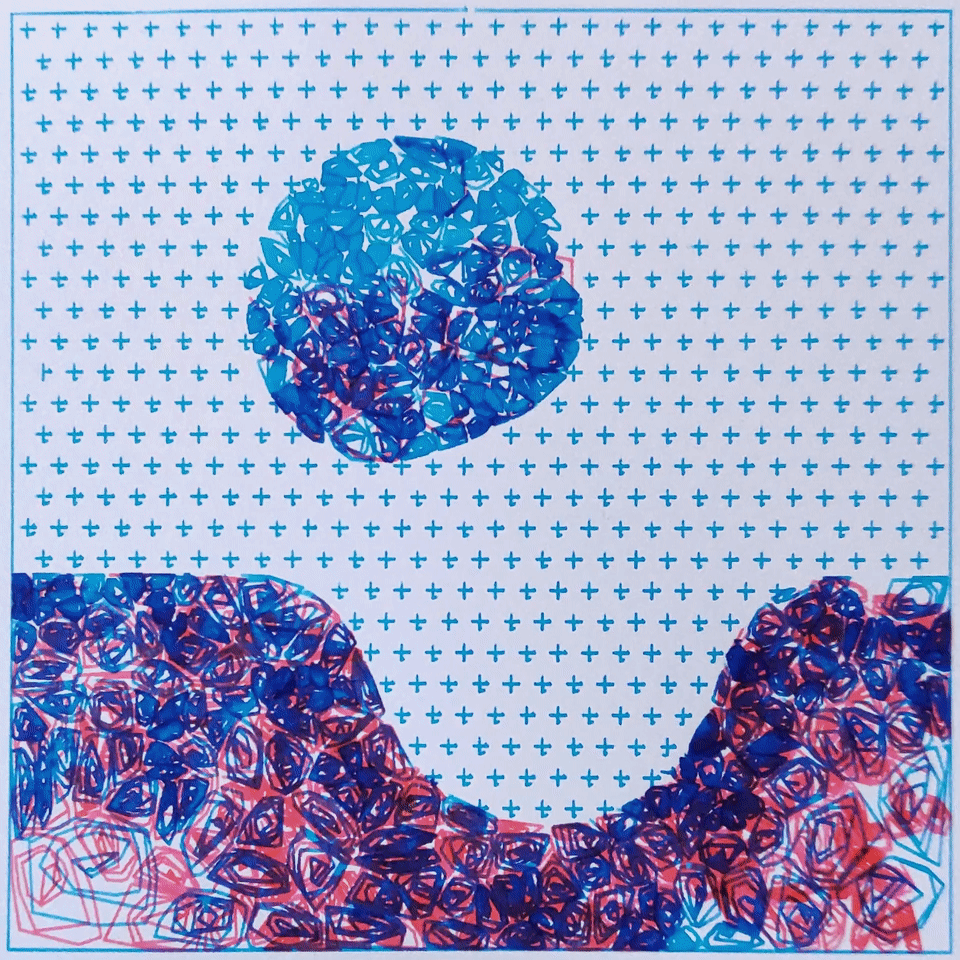Here is "Jumping blob" my second "plot loop" (see article). The main digital art is a 1920p video loop of 8 frames available as a Tezos hicetnunc NFT. The physical art is 8 frames of A5 size, the square of the drawing is 12cm by 12cm and they are offered when buying the NFT (8 editions, assigned in buy order).

There are 8 frames plotted recreating the "jumping blob" animation (shader implemented in https://greweb.me/shaderday/67). Each frame is plotted with two fountain pens (Diamine inks: Pink and Turquoise) on Canson Bristal (A5 format), and takes about an hour to plot.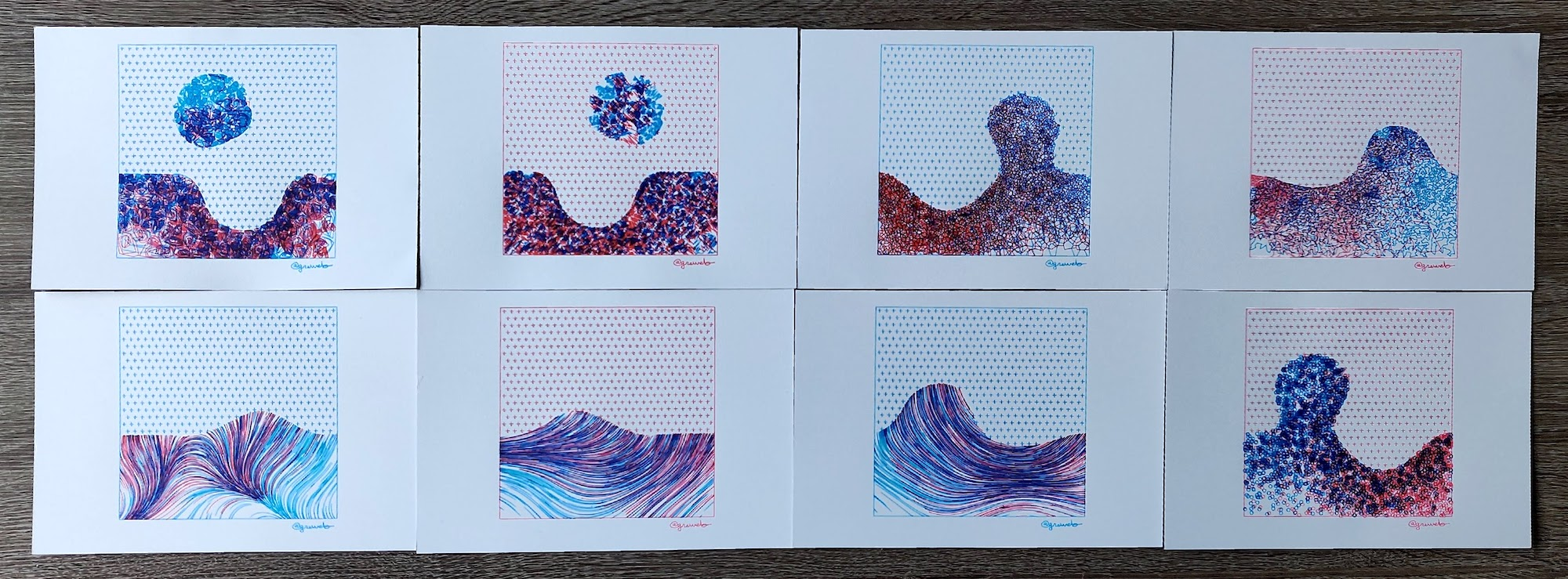Each frame revisit a specific technique that I explored in the past months:

• Frame 1: Voronoi distribution + samples spiral
• Frame 2: Voronoi distribution + samples sorted
• Frame 3: Voronoi polygons
• Frame 4: Voronoi distribtion + TSP
• Frame 5: sampling points and starting lines with vector field (low frequency)
• Frame 6: sampling points and starting lines with vector field (aligned horizontally)
• Frame 7: sampling points and starting lines with vector field (more curvy)
• Frame 8: circles plotting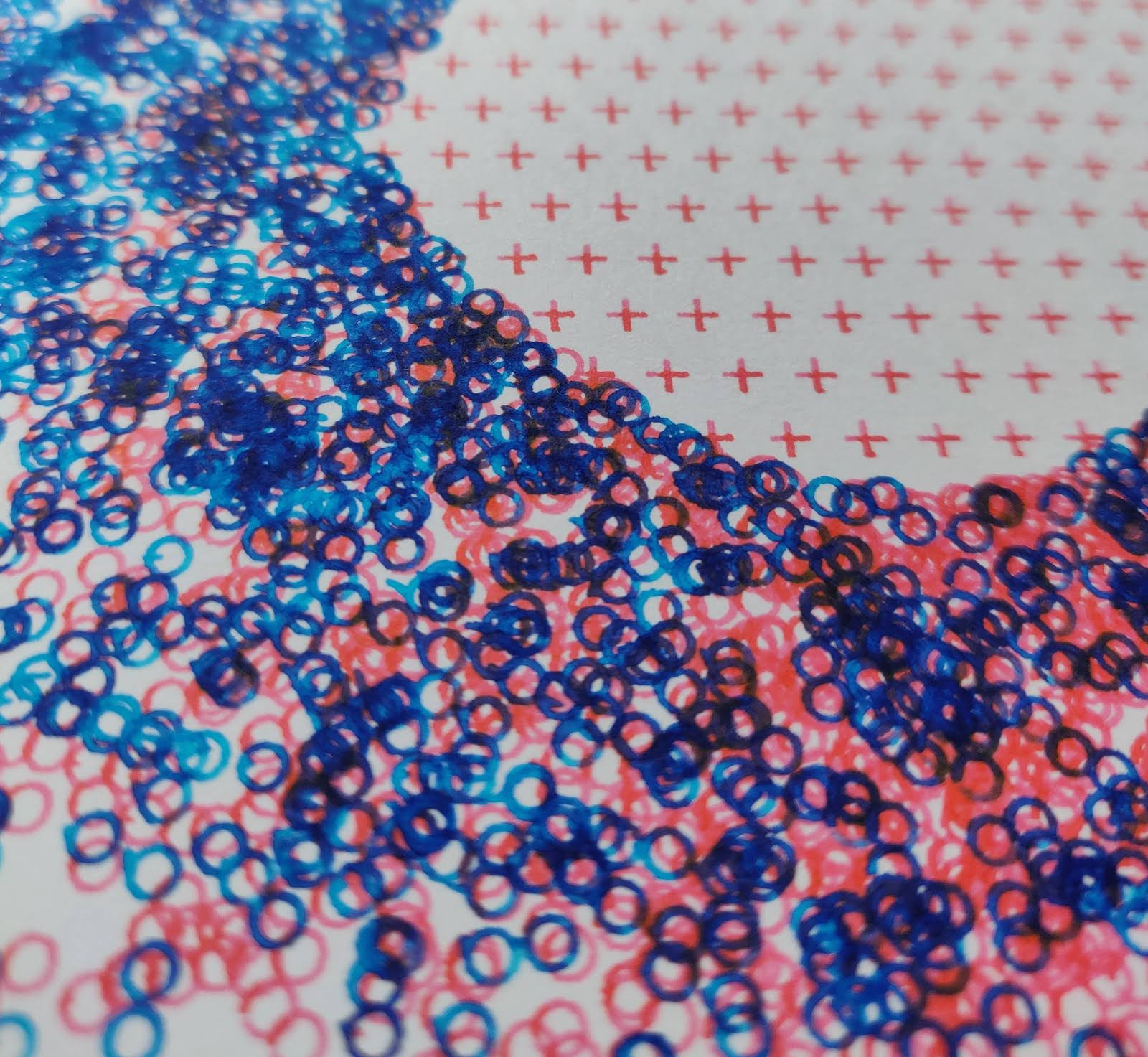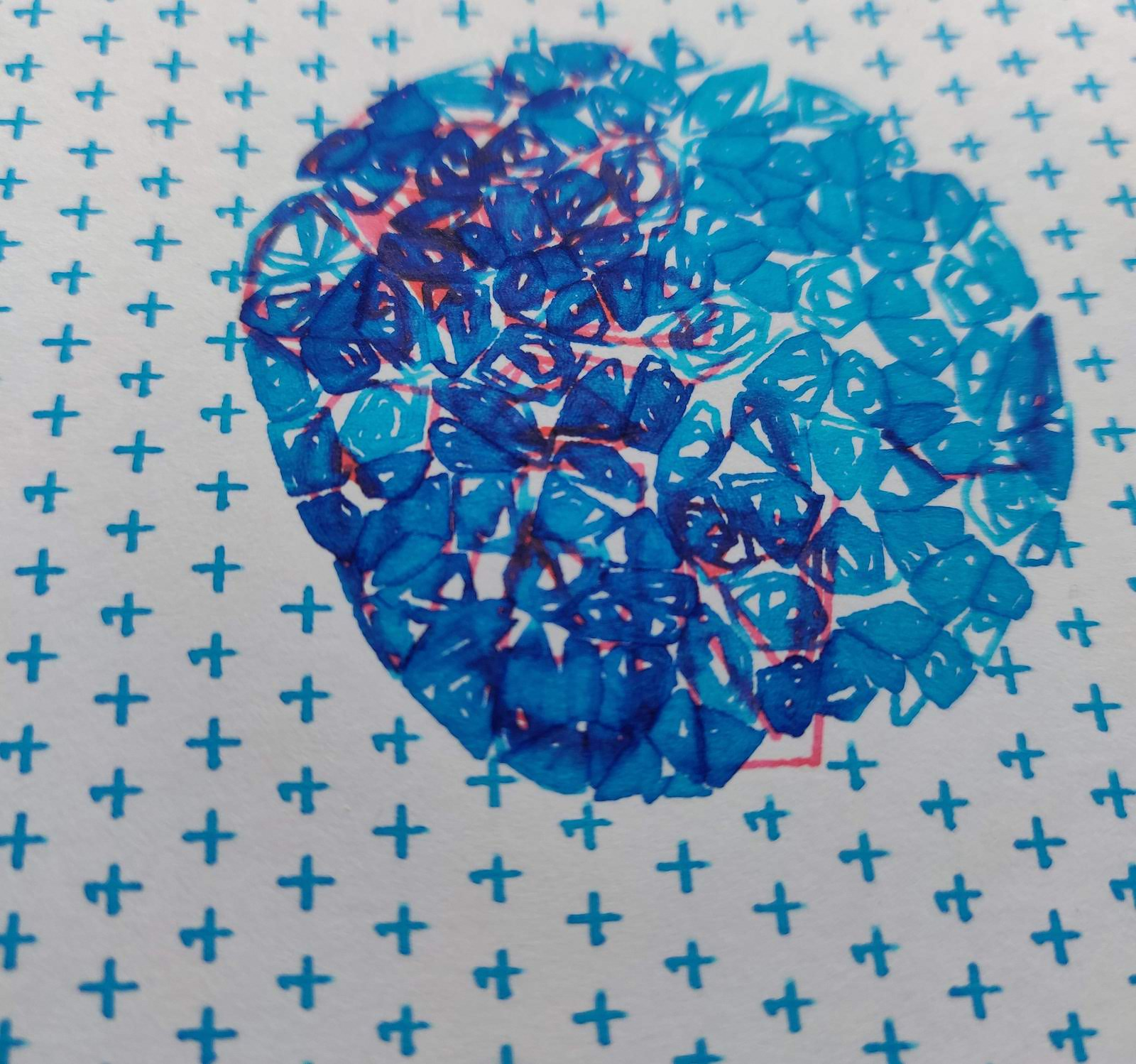The generator was completely reimplemented, including the "scene" itself which is a port of the GLSL code into Rustlang with some adjustments (two different colors are spread on different areas):

``````fn jumping_blob(f: f64, o: (f64, f64)) -> Vec<f64> {
let mut p = o;
let bezier = Bezier::new(0.0, 0.1, 1.0, 0.9);
let x = bezier.calculate(f as f32) as f64;
let t = x * 2. * PI;
let smoothing = 0.15;
let dist = 0.2;
p.0 -= 0.5;
p.1 -= 0.5;
p.1 *= -1.0;
p = p_r(p, PI / 2.0);
let q = p;
p = p_r(p, -t);
let s = f_op_difference_round(
f_op_union_round(
q.0.max(0.1 + q.0),
length((p.0 + dist, p.1)) - radius,
smoothing,
),
length((p.0 - dist, p.1)) - radius,
smoothing,
);
let v = smoothstep(-0.6, 0.0, s).powf(2.0)
* (if s < 0.0 { 1.0 } else { 0.0 });
vec![
v * (0.001 + smoothstep(-0.5, 1.5, p.0)),
v * (0.001 + smoothstep(1.5, -0.5, p.0)),
]
}
fn p_r(p: (f64, f64), a: f64) -> (f64, f64) {
(
a.cos() * p.0 + a.sin() * p.1,
a.cos() * p.1 - a.sin() * p.0,
)
}
fn length(l: (f64, f64)) -> f64 {
(l.0 * l.0 + l.1 * l.1).sqrt()
}
fn f_op_union_round(a: f64, b: f64, r: f64) -> f64 {
r.max(a.min(b))
- length(((r - a).max(0.), (r - b).max(0.)))
}
fn f_op_intersection_round(a: f64, b: f64, r: f64) -> f64 {
(-r).min(a.max(b))
+ length(((r + a).max(0.), (r + b).max(0.)))
}
fn f_op_difference_round(a: f64, b: f64, r: f64) -> f64 {
f_op_intersection_round(a, -b, r)
}
``````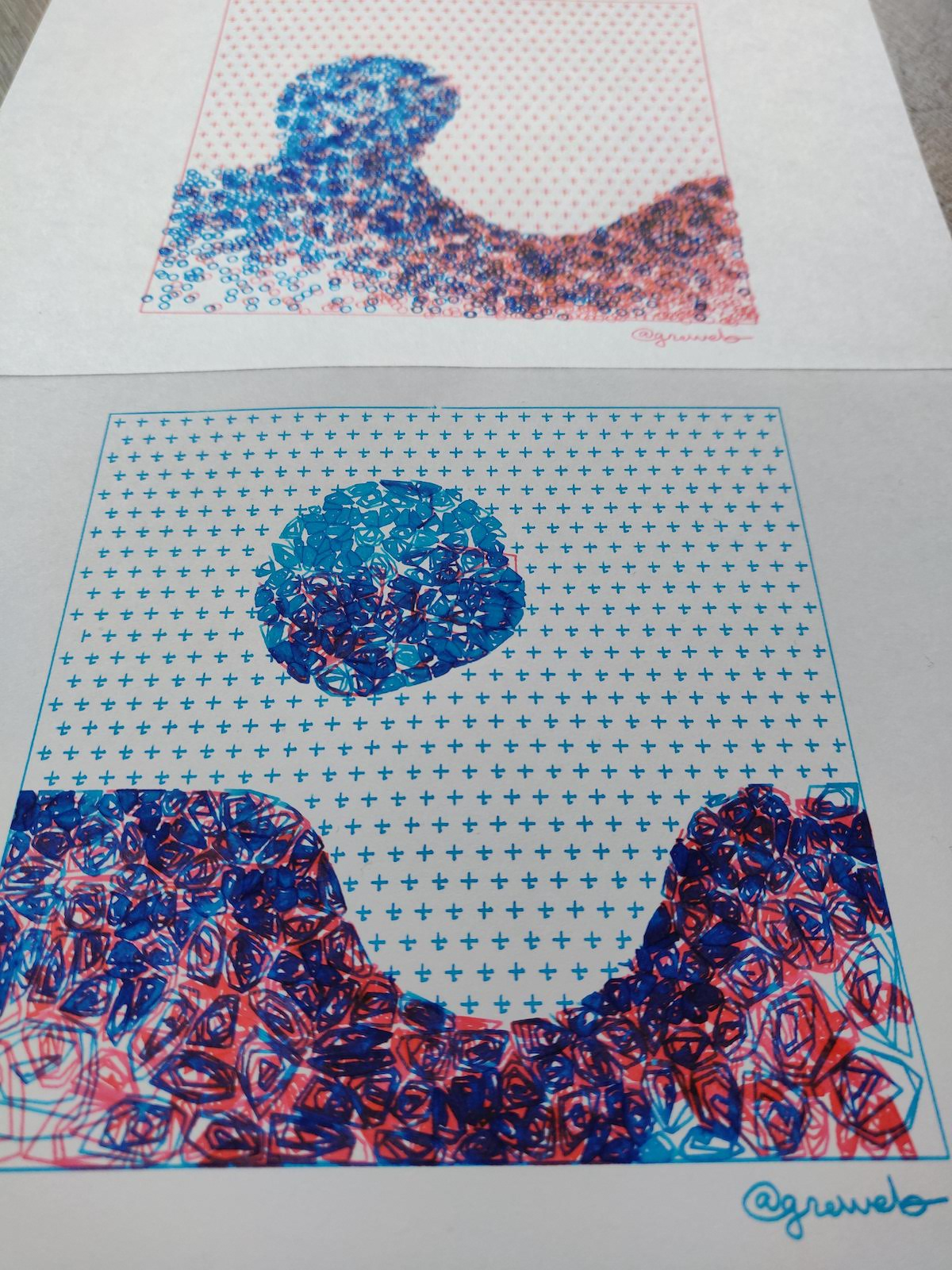It's one of the first time I try to work on the "scene composition" and I've also used a pattern filled with "+" for the background. I want to explore more of these in the future.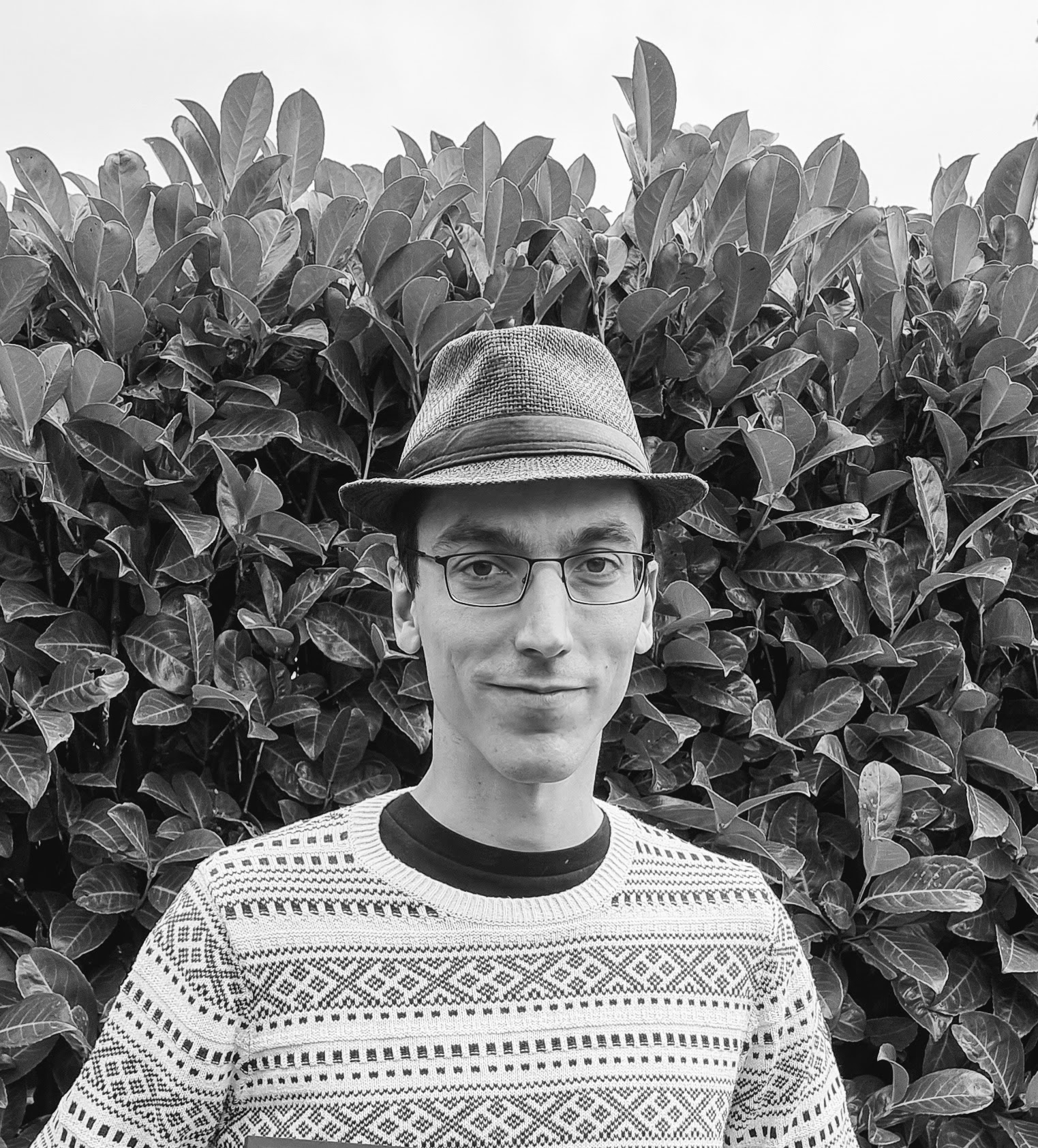creative coder experimenting with GLSL shaders, Rust, and fountain pens robot plots. infinite noise explorer.